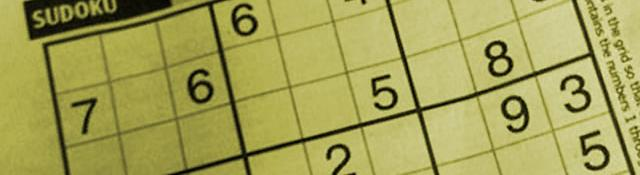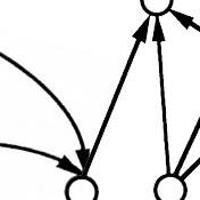## Extracting the grid

Series: SuDoKu Grabber in OpenCV:

1. The Plot
2. Grid detection
3. Extracting the grid
4. Extracting digits
5. Recognizing digits

In the last post, we had found some lines. But the numerous lines were not good enough for detecting the location of the puzzle. So we'll do some math today and find out exactly where the puzzle is. We'll also un-distort the puzzle so we have a perfect top-down view of the sudoku puzzle.

## Merging lines

Each physical line on the image has several "mathematical" lines associated with it. This is because of its One way to fix this is to "merge" lines that are close by.

By merging lines I mean averaging nearby lines. So lines that are within a certain distance will "fuse" together.

We'll write another function to fuse lines together.  Start off by:

```void mergeRelatedLines(vector<Vec2f> *lines, Mat &img)
{
vector<Vec2f>::iterator current;
for(current=lines->begin();current!=lines->end();current++)
{
```

The iterator helps traverse the array list. Each element of the list contains 2 things: rho and theta (the normal form of a line).

During the merging process, certain lines will fuse together. So, we'll need to mark lines that have been fused (so they aren't considered for other things). This is done by setting the rho to zero and theta to -100 (an impossible value). So whenever we encounter such a line, we simply skip it:

```if((*current)==0 && (*current)==-100) continue;
```

Now, we store the rho and theta for the current line in two variables:

```        float p1 = (*current);
float theta1 = (*current);
```

With these two values, we find two points on the line:;

```        Point pt1current, pt2current;
if(theta1>CV_PI*45/180 && theta1<CV_PI*135/180)
{
pt1current.x=0;

pt1current.y = p1/sin(theta1);

pt2current.x=img.size().width;
pt2current.y=-pt2current.x/tan(theta1) + p1/sin(theta1);
}
else
{
pt1current.y=0;

pt1current.x=p1/cos(theta1);

pt2current.y=img.size().height;
pt2current.x=-pt2current.y/tan(theta1) + p1/cos(theta1);

}
```

If the is horizontal (theta is around 90 degrees), we find a point at the extreme left (x=0) and one at the extreme right (x=img.width). If not, we find a point at the extreme top (y=0) and one at extreme bottom (y=img.height).

All the calculations are done based on the normal form of a line.

Next, we start iterating over the lines again:

```        vector<Vec2f>::iterator    pos;
for(pos=lines->begin();pos!=lines->end();pos++)
{
if(*current==*pos) continue;
```

With this loop, we can compare every line with every other line. If we find that the line current is the same as the line pos, we continue. No point fusing the same line.

Now we check if the lines are within a certain distance of each other:

```            if(fabs((*pos)-(*current))<20 && fabs((*pos)-(*current))<CV_PI*10/180)
{
float p = (*pos);
float theta = (*pos);
```

If they are, we store the rho and theta for the line pos.

And again, we find two points on the line pos:

```                Point pt1, pt2;
if((*pos)>CV_PI*45/180 && (*pos)<CV_PI*135/180)
{
pt1.x=0;
pt1.y = p/sin(theta);
pt2.x=img.size().width;
pt2.y=-pt2.x/tan(theta) + p/sin(theta);
}
else
{
pt1.y=0;
pt1.x=p/cos(theta);
pt2.y=img.size().height;
pt2.x=-pt2.y/tan(theta) + p/cos(theta);
}
```

Now if endpoints of the line pos and the line current are close to each other, we fuse them:

```                if(((double)(pt1.x-pt1current.x)*(pt1.x-pt1current.x) + (pt1.y-pt1current.y)*(pt1.y-pt1current.y)<64*64) &&
((double)(pt2.x-pt2current.x)*(pt2.x-pt2current.x) + (pt2.y-pt2current.y)*(pt2.y-pt2current.y)<64*64))
{
// Merge the two
(*current) = ((*current)+(*pos))/2;

(*current) = ((*current)+(*pos))/2;

(*pos)=0;
(*pos)=-100;
}
```

That's all there is to fusing lines:

```            }
}
}
}
```

Now you can add a call to this function after the Hough transform:

```    vector<Vec2f> lines;

HoughLines(outerBox, lines, 1, CV_PI/180, 200);

mergeRelatedLines(&lines, sudoku); // Add this line
```

And thus, we'll have merged neighbouring lines.

## Finding extreme lines

Now we'll try and detect lines that are nearest to the top edge, bottom edge, right edge and the left edge. These will be the outer boundaries of the sudoku puzzle. We start by adding these lines after the mergeRelatedLines call:

```    // Now detect the lines on extremes
Vec2f topEdge = Vec2f(1000,1000);    double topYIntercept=100000, topXIntercept=0;
Vec2f bottomEdge = Vec2f(-1000,-1000);        double bottomYIntercept=0, bottomXIntercept=0;
Vec2f leftEdge = Vec2f(1000,1000);    double leftXIntercept=100000, leftYIntercept=0;
Vec2f rightEdge = Vec2f(-1000,-1000);        double rightXIntercept=0, rightYIntercept=0;
```

The initial values of each edge is initially set to a ridiculous value. This will ensure it gets to the proper edge later on. Now we loop over all lines:

```    for(int i=0;i<lines.size();i++)
{

Vec2f current = lines[i];

float p=current;

float theta=current;

if(p==0 && theta==-100)
continue;
```

We store the rho and theta values. If we encounter a "merged" line, we simply skip it. Now we use the normal form of line to calculate the x and y intercepts (the place where the lines intersects the X and Y axis)

```        double xIntercept, yIntercept;
xIntercept = p/cos(theta);
yIntercept = p/(cos(theta)*sin(theta));
```

If the line is nearly vertical:

```        if(theta>CV_PI*80/180 && theta<CV_PI*100/180)
{
if(p<topEdge)

topEdge = current;

if(p>bottomEdge)
bottomEdge = current;
}
```

Otherwise, if they are nearly horizontal,

```        else if(theta<CV_PI*10/180 || theta>CV_PI*170/180)
{
if(xIntercept>rightXIntercept)
{
rightEdge = current;
rightXIntercept = xIntercept;
}
else if(xIntercept<=leftXIntercept)
{
leftEdge = current;
leftXIntercept = xIntercept;
}
}
}
```

We've ignored any lines that have slopes at other angles. And this also ends the loop. Now, at the end of this loop, we'll have the extreme lines. Just for visualizing it, we'll draw those lines on the original image:

```    drawLine(topEdge, sudoku, CV_RGB(0,0,0));
drawLine(bottomEdge, sudoku, CV_RGB(0,0,0));
drawLine(leftEdge, sudoku, CV_RGB(0,0,0));
drawLine(rightEdge, sudoku, CV_RGB(0,0,0));
```

Next, we'll calculate the intersections of these four lines. First, we find two points on each line. Then using some math, we can calculate exactly where any two particular lines intersect:

```    Point left1, left2, right1, right2, bottom1, bottom2, top1, top2;

int height=outerBox.size().height;

int width=outerBox.size().width;

if(leftEdge!=0)
{
left1.x=0;        left1.y=leftEdge/sin(leftEdge);
left2.x=width;    left2.y=-left2.x/tan(leftEdge) + left1.y;
}
else
{
left1.y=0;        left1.x=leftEdge/cos(leftEdge);
left2.y=height;    left2.x=left1.x - height*tan(leftEdge);

}

if(rightEdge!=0)
{
right1.x=0;        right1.y=rightEdge/sin(rightEdge);
right2.x=width;    right2.y=-right2.x/tan(rightEdge) + right1.y;
}
else
{
right1.y=0;        right1.x=rightEdge/cos(rightEdge);
right2.y=height;    right2.x=right1.x - height*tan(rightEdge);

}

bottom1.x=0;    bottom1.y=bottomEdge/sin(bottomEdge);

bottom2.x=width;bottom2.y=-bottom2.x/tan(bottomEdge) + bottom1.y;

top1.x=0;        top1.y=topEdge/sin(topEdge);
top2.x=width;    top2.y=-top2.x/tan(topEdge) + top1.y;
```

The code above finds two points on a line. The right and left edges need the "if" construct. These edges are vertical. They can have infinite slope, something a computer cannot represent. So I check if they have infinite slope or not. If it does, calculate two points using a "safe" method. Otherwise, the normal method can be used.

Now this part calculates the actual intersection points:

```     // Next, we find the intersection of  these four lines
double leftA = left2.y-left1.y;
double leftB = left1.x-left2.x;

double leftC = leftA*left1.x + leftB*left1.y;

double rightA = right2.y-right1.y;
double rightB = right1.x-right2.x;

double rightC = rightA*right1.x + rightB*right1.y;

double topA = top2.y-top1.y;
double topB = top1.x-top2.x;

double topC = topA*top1.x + topB*top1.y;

double bottomA = bottom2.y-bottom1.y;
double bottomB = bottom1.x-bottom2.x;

double bottomC = bottomA*bottom1.x + bottomB*bottom1.y;

// Intersection of left and top
double detTopLeft = leftA*topB - leftB*topA;

CvPoint ptTopLeft = cvPoint((topB*leftC - leftB*topC)/detTopLeft, (leftA*topC - topA*leftC)/detTopLeft);

// Intersection of top and right
double detTopRight = rightA*topB - rightB*topA;

CvPoint ptTopRight = cvPoint((topB*rightC-rightB*topC)/detTopRight, (rightA*topC-topA*rightC)/detTopRight);

// Intersection of right and bottom
double detBottomRight = rightA*bottomB - rightB*bottomA;
CvPoint ptBottomRight = cvPoint((bottomB*rightC-rightB*bottomC)/detBottomRight, (rightA*bottomC-bottomA*rightC)/detBottomRight);// Intersection of bottom and left
double detBottomLeft = leftA*bottomB-leftB*bottomA;
CvPoint ptBottomLeft = cvPoint((bottomB*leftC-leftB*bottomC)/detBottomLeft, (leftA*bottomC-bottomA*leftC)/detBottomLeft);
```

Now we have the points. Now we can correct the skewed perspective. First, we find the longest edge of the puzzle. The new image will be a square of the length of the longest edge.

```    int maxLength = (ptBottomLeft.x-ptBottomRight.x)*(ptBottomLeft.x-ptBottomRight.x) + (ptBottomLeft.y-ptBottomRight.y)*(ptBottomLeft.y-ptBottomRight.y);
int temp = (ptTopRight.x-ptBottomRight.x)*(ptTopRight.x-ptBottomRight.x) + (ptTopRight.y-ptBottomRight.y)*(ptTopRight.y-ptBottomRight.y);

if(temp>maxLength) maxLength = temp;

temp = (ptTopRight.x-ptTopLeft.x)*(ptTopRight.x-ptTopLeft.x) + (ptTopRight.y-ptTopLeft.y)*(ptTopRight.y-ptTopLeft.y);

if(temp>maxLength) maxLength = temp;

temp = (ptBottomLeft.x-ptTopLeft.x)*(ptBottomLeft.x-ptTopLeft.x) + (ptBottomLeft.y-ptTopLeft.y)*(ptBottomLeft.y-ptTopLeft.y);

if(temp>maxLength) maxLength = temp;

maxLength = sqrt((double)maxLength);
```

Simple code. We calculate the length of each edge. Whenever we find a longer edge, we store its length squared. And finally when we have the longest edge, we do a square root to get its exact length.

Next, we create source and destination points:

```Point2f src, dst;
src = ptTopLeft;            dst = Point2f(0,0);
src = ptTopRight;        dst = Point2f(maxLength-1, 0);
src = ptBottomRight;        dst = Point2f(maxLength-1, maxLength-1);
src = ptBottomLeft;        dst = Point2f(0, maxLength-1);
```

The top left point in the source is equivalent to the point (0,0) in the corrected image. And so on.

Then we create a new image and do the undistortion:

```Mat undistorted = Mat(Size(maxLength, maxLength), CV_8UC1);
cv::warpPerspective(original, undistorted, cv::getPerspectiveTransform(src, dst), Size(maxLength, maxLength));
```

Now the image undistorted has the corrected image. Simple as that!

## Summary

This was a long one! In this post, we merged lines that could possibly be representing the same physical border. Then we found lines nearest to the edges. Then, we calculated intersections. And finally, we undistorted the puzzle.

The next step is recognizing the characters and generating an internal representation of the puzzle. Then, we can solve the puzzle finally!

## More in the series

This tutorial is part of a series called SuDoKu Grabber in OpenCV:

1. The Plot
2. Grid detection
3. Extracting the grid
4. Extracting digits
5. Recognizing digits

#### Related posts#### 78xx voltage regulator#### 7 unique neural network architectures#### Community Core Vision: An app for simple vision stuff

Utkarsh Sinha created AI Shack in 2010 and has since been working on computer vision and related fields. He is currently at Microsoft working on computer vision.